Author: Oscar Cronquist Article last updated on February 13, 2023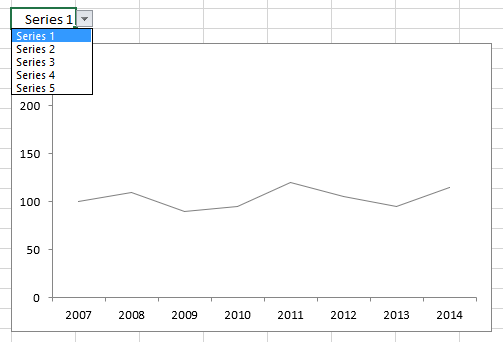This article demonstrates how to create an animation using a line chart in Excel. The user selects a series in a drop-down box and a macro animates the chart.

The animated image below shows what happens when the user selects a series, as you can see the chart has a small trailing shadow effect.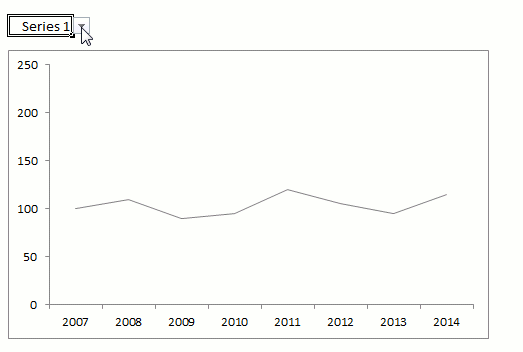### How I made this chart

The chart data is in cell range B3:J8. A small VBA macro calculates the difference between the current series and the new series and then divides it by 20. The result is shown in cell range C10:J10.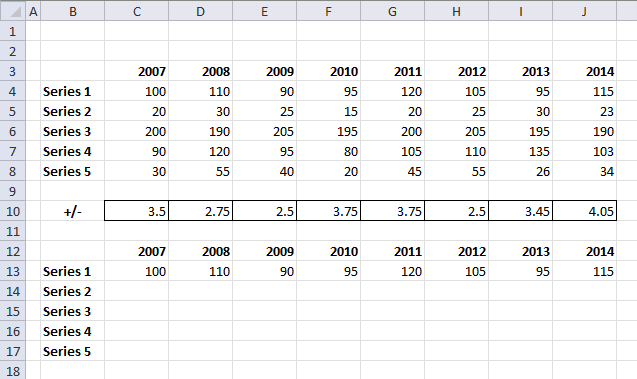The chart data source is cell range C13:J17. The chart uses 4 lines to create the trailing shadow effect. Series 1 is the main line, the others are shadows.

Here you can see the calculations in action.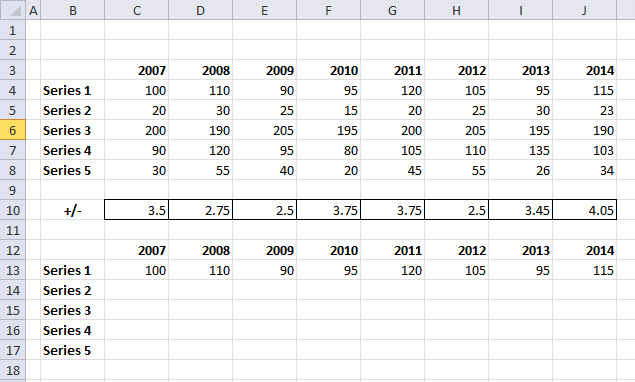### Event code

```'Event code that fires as soon as any cell value in the worksheet has changed
Private Sub Worksheet_Change(ByVal Target As Range)

'If ... then statement - Check if target cell adress is equal to L6

'Enable error handling
On Error Resume Next

'Find position of value saved cell L6 in cell range B4:B8 and save to variable x
x = WorksheetFunction.Match(Range("L6"), Range("B4:B8"), 0) - 1

'Stop macro if an error has occurred
If Err > 0 Then Exit Sub

'Disable error handling
On Error GoTo 0

'For ... Next statement- Iterate from 1 to 8 using variable i
For i = 1 To 8

'Calculate and save value from data table to cell range C13:J13
Range("C10:J10").Cells(i) = (Range(Cells(4 + x, "C"), Cells(4 + x, "J")).Cells(i) - _
Range("C13:J13").Cells(i)) / 20
Next i

'For ... Next statement- Iterate from 1 to 20 using variable i
For i = 1 To 20

'Save values in cell range C13:J16 to cell range C14:J17
Range("C14:J17") = Range("C13:J16").Value

'For ... Next statement- Iterate from 1 to 8 using variable j
For j = 1 To 8

'Add cell value from cell range C13:J13 and C10:J10 and save to cell range C13:J13 based on variable j
Range("C13:J13").Cells(j) = Range("C13:J13").Cells(j) + Range("C10:J10").Cells(j)
Next j

'Show changes on screen
DoEvents

'And once again to make it work in Excel 365
DoEvents
Next i
For j = 1 To 4
Range("C14:J17") = Range("C13:J16").Value
Range("C14:J14") = ""
DoEvents
Next j
End If
End Sub
```

### Where to put the code?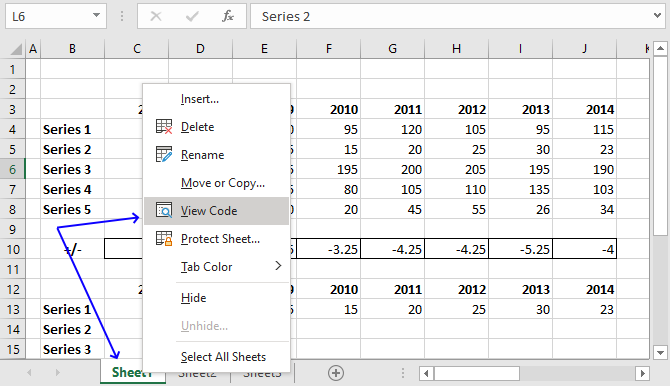1. Righ-press with left mouse button on the worksheet tab located on the bottom left corner, see image above. A pop-up menu appears.
2. Press with mouse on "View Code".
3. The Visual Basic Editor opens up with the corresponding worksheet module open.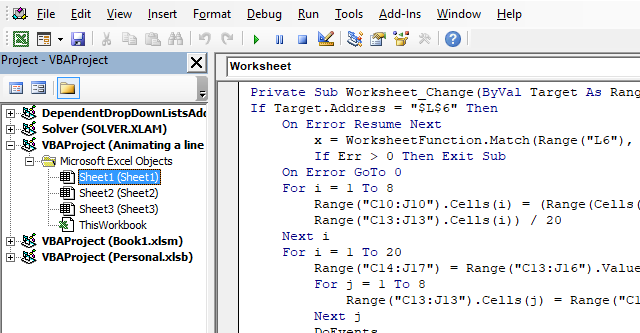4. Copy VBA code above.
5. Paste VBA code to the worksheet module.
Note, save the workbook using file extension *.xlsm (macro-enabled workbook) to attach the VBA code to the file.